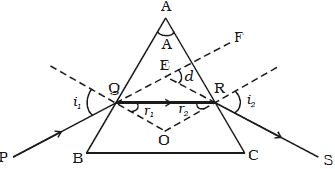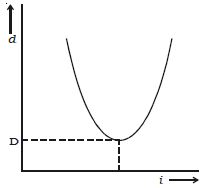# Explain Refraction of Light through a Prism

Refraction of light through a prism:

The figure shows the cross-section of a triangular prism ABC, placed in air. Let ‘A’ be the refracting angle of the prism. A ray of light PQ incident on the refracting face AB. gets refracted along QR and emerges along RS.Fig: refraction through a prism

The angle of incidence and refraction at the two faces are i1, r1, r2 and i2 respectively. The angle between the incident ray PQ and the emergent ray RS is called angle of deviation, d.

In the ∆QER, the exterior angle FER = angle EQR + angle ERQ

d = (i1– r1) + (i2– r2)

so, d = (i1 + i2) – (r1 + r2) … … … (1)

In the quadrilateral AQOR, the angles at Q and R are right angles

angle Q + angle R = 1800

so, A + angle QOR = 1800 … … … (2)

also from the ∆QOR;

r1 + r2 + angle QOR = 1800 … … … (3)

From equation (2) and (3), r1 + r2 = A … … … (4)

Substituting in equation (1)

A + d = i1 + i2 … … … (5)

For a given prism and for a light of given wavelength. the angle of deviation depends upon the angle of incidence.

As the angle of incidence, I gradually increase the angle of deviation d decreases, reaches a minimum value D and then increases. D is called the angle of minimum deviation. It will be seen from the graph (Figure) that there is only one angle of incidence for which the deviation is a minimum.At minimum deviation position, the incident ray and emergent ray are symmetric with respect to the base of the prism. (i.e) the refracted ray QR is parallel to the base of the prism.

At the minimum deviation, i1 = i2 = i; and r1 = r2 = r

From equation (4), 2r = A or, r = A/2

and from equation (5), 2i = A+D or, i = (A+D)/2

The refractive index is, μ = [sin i / sin r]

so, μ = [sin (A+D)/2] / sin (A/2)